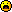﻿ Unreal Software - Thread: Random maps+maptypes

# ForumCS2DScriptsRandom maps+maptypes

#Random maps+maptypes

10 replies
Goto Page1Suprise
BANNED
OfflineHi again guyz. I need a scriptSo. There is list of maps
Ex: Map_list: "mymap1","mymap2",balbalabla

There is a mapchange in every 1 hour:
secssa = 0
Code:
1
2
3
4
5
6
7
8
9
function autotextf()
secssa = secssa + 1
if secssa >= 3590 then
msg("©255000255NEXT MAP WILL BE CHANGED IN 10 SECONDS!")
--This is what I want!
secssa = 0
end
end

Timer is started: timer(10000,"MORE here!
and the random map is changing on server from Map_list!

Sorry if you dont understand Dx
edited 1×, last 17.04.12 04:21:24 pm
Cure Pikachu
User
OfflineMaybe something like this.Code:
1
2
3
4
5
6
7
8
9
10
11
12
13
14
15
16
secssa = 0

-- Feel free to modify this table, but leave the variable name as it is
maps = {"de_dust","de_dust2","de_aztec","de_cs2d","cs_office"}

function autotextf()
secssa = secssa + 1
if secssa == 3590 then
msg("©255000255NEXT MAP WILL BE CHANGED IN 10 SECONDS!")
end
if secssa == 3600 then
local r = math.random(1,#maps) -- RNG
parse("map "..maps[r])
end
endSuprise
BANNED
OfflineYes it is:D
Thanks you man. you are always helping meEDIT: I dont want to create new soo here is my new question.
Maptypes script

If maptype zm_ then
My Code
if maptype ctf_ then
My code
Cure Pikachu
User
Offline@Suprise
Well, here's the "prototype" code. This checks the maptype by its prefix. (Updated)
Code:
1
2
3
4
5
6
7
8
9
10
11
12
13
14
15
16
17
-- Function
function prefix(n)
local x = tonumber(string.len(n))
if string.sub(map("name"),1,x) == n then
return true
end
return false
end

-- Examples
if prefix("ctf_") then -- If current map is a CTF map
-- Do something
end

if prefix("zm_") then -- If current map is a ZM map
-- Do something
end

Include the underscore, otherwise it won't be accurate!
edited 2×, last 17.04.12 06:46:34 pmSuprise
BANNED
Offline-- Function
function prefix(n)
local mapname = map("name")
local end = string.len(n)
if string.lower(string.sub(mapname,1,end)) == n then
return true
end
return false
end

Something wrong on 4th line.
Cure Pikachu
User
OfflineCode:
1
local end = tonumber(string.len(n))

That might do the trick.

EDIT:
Never mind, I screwed up my variable names (end is reserved), so the code in my previous post is updated.Avo 's code would work just as well though.
edited 3×, last 17.04.12 06:47:12 pmAvo
User
OfflineCode:
1
2
3
4
5
6
7
8
9
10
function check()
local map=map("name")
if map:sub(1,3)=="zm_" then
--your code here
elseif map:sub(1,3)=="dm_" then
--your code here
elseif map:sub(1,4)=="ctf_" then
--your code here
end
end

should works
Suprise
BANNED
OfflineCure Pikachu has written:
Code:
1
local end = tonumber(string.len(n))

That might do the trick.
EDIT: Never mind, I screwed up my variable names.Avo 's code would work just as well though.

Dont work@Avo: Now testing itEDIT: no workAvo
User
Offlinedoesn't work? did you add addhook to function check?

Code:
1
2
3
4
5
6
7
8
9
10
11
function check()
local map=map("name")
if map:sub(1,3)=="zm_" then
--your code here
elseif map:sub(1,3)=="dm_" then
--your code here
elseif map:sub(1,4)=="ctf_" then
--your code here
end
end

?Any errors in console?
Suprise
BANNED
OfflineAvo has written:doesn't work? did you add addhook to function check?

Code:
1
2
3
4
5
6
7
8
9
10
11
function check()
local map=map("name")
if map:sub(1,3)=="zm_" then
--your code here
elseif map:sub(1,3)=="dm_" then
--your code here
elseif map:sub(1,4)=="ctf_" then
--your code here
end
end

?Any errors in console?

I added but in console nothing wrong there.EDIT: Pikuachi. your code is work:
-- Function
function prefix(n)
local x = tonumber(string.len(n))
if string.sub(map("name"),1,x) == n then
return true
end
return false
end

-- Examples
if prefix("ctf_") then -- If current map is a CTF map
-- Do something
end

if prefix("zm_") then -- If current map is a ZM map
-- Do something
end

Thanks you very muchAvo
User
OfflineCode:
1
2
3
4
5
6
7
8
9
10
11
function check()
local map=map("name")
if map:sub(1,3)=="zm_" then
msg("lua test: its zm map@C")
elseif map:sub(1,3)=="dm_" then
msg("lua test: its dm map @C")
elseif map:sub(1,4)=="ctf_" then
msg("lua test: its ctf map @C")
end
end

I tested. Works.1﻿